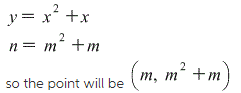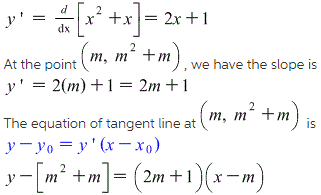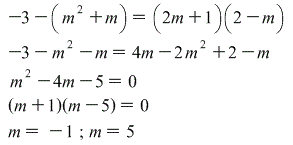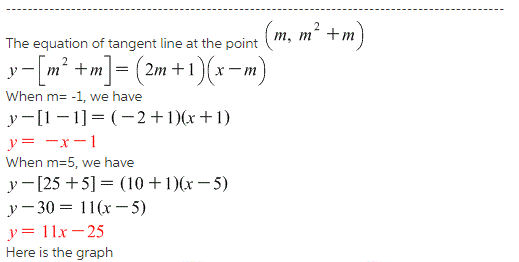# Find equations of both lines through the point (2,-3) that are tangent to the parabola y = x2 + x

Find equations of both lines through the point (2,-3) that are tangent to the parabola y = x2 + x.
y = …(smaller slope)
y = …(larger slope)